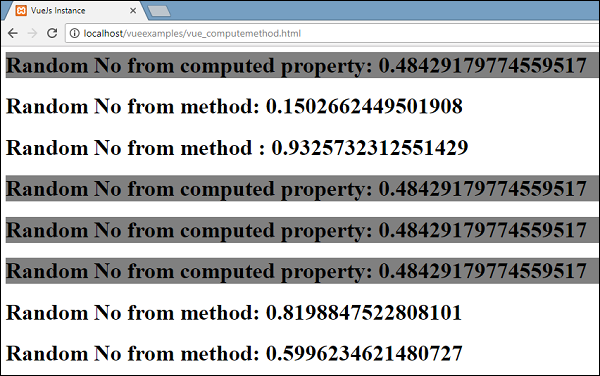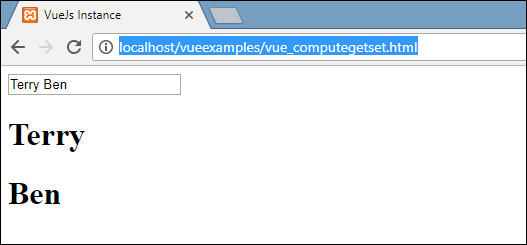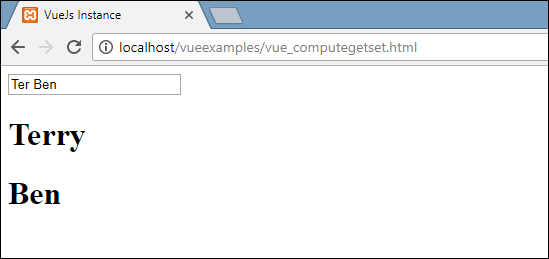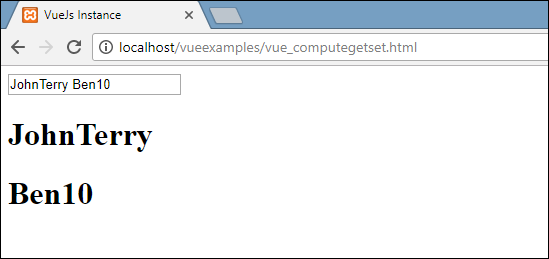# VueJS - Computed Properties

We have already seen methods for Vue instance and for components. Computed properties are like methods but with some difference in comparison to methods, which we will discuss in this chapter.

At the end of this chapter, we will be able to make a decision on when to use methods and when to use computed properties.

Let’s understand computed properties using an example.

### Example

```<html>
<title>VueJs Instance</title>
<script type = "text/javascript" src = "js/vue.js"></script>
<body>
<div id = "computed_props">
FirstName : <input type = "text" v-model = "firstname" /> <br/><br/>
LastName : <input type = "text" v-model = "lastname"/> <br/><br/>
<h1>My name is {{firstname}} {{lastname}}</h1>
<h1>Using computed method : {{getfullname}}</h1>
</div>
<script type = "text/javascript" src = "js/vue_computedprops.js"></script>
</body>
</html>
```

vue_computeprops.js

```var vm = new Vue({
el: '#computed_props',
data: {
firstname :"",
lastname :"",
birthyear : ""
},
computed :{
getfullname : function(){
return this.firstname +" "+ this.lastname;
}
}
})
```

Here, we have created .html file with firstname and lastname. Firstname and Lastname is a textbox which are bound using properties firstname and lastname.

We are calling the computed method getfullname, which returns the firstname and the lastname entered.

```computed :{
getfullname : function(){
return this.firstname +" "+ this.lastname;
}
}
```

When we type in the textbox the same is returned by the function, when the properties firstname or lastname is changed. Thus, with the help of computed we don’t have to do anything specific, such as remembering to call a function. With computed it gets called by itself, as the properties used inside changes, i.e. firstname and lastname.

The same is displayed in the following browser. Type in the textbox and the same will get updated using the computed function.Now, let’s try to understand the difference between a method and a computed property. Both are objects. There are functions defined inside, which returns a value.

In case of method, we call it as a function, and for computed as a property. Using the following example, let us understand the difference between method and computed property.

```<html>
<title>VueJs Instance</title>
<script type = "text/javascript" src = "js/vue.js"></script>
<body>
<div id = "computed_props">
<h1 style = "background-color:gray;">Random No from computed property: {{getrandomno}}</h1>
<h1>Random No from method: {{getrandomno1()}}</h1>
<h1>Random No from method : {{getrandomno1()}}</h1>
<h1  style = "background-color:gray;">Random No from computed property: {{getrandomno}}</h1>
<h1  style = "background-color:gray;">Random No from computed property: {{getrandomno}}</h1>
<h1  style = "background-color:gray;">Random No from computed
property: {{getrandomno}}</h1>
<h1>Random No from method: {{getrandomno1()}}</h1>
<h1>Random No from method: {{getrandomno1()}}</h1>
</div>
<script type = "text/javascript">
var vm = new Vue({
el: '#computed_props',
data: {
name : "helloworld"
},
methods: {
getrandomno1 : function() {
return Math.random();
}
},
computed :{
getrandomno : function(){
return Math.random();
}
}
});
</script>
</body>
</html>
```

In the above code, we have created a method called getrandomno1 and a computed property with a function getrandomno. Both are giving back random numbers using Math.random().

It is displayed in the browser as shown below. The method and computed property are called many times to show the difference.If we look at the values above, we will see that the random numbers returned from the computed property remains the same irrespective of the number of times it is called. This means everytime it is called, the last value is updated for all. Whereas for a method, it’s a function, hence, everytime it is called it returns a different value.

## Get/Set in Computed Properties

In this section, we will learn about get/set functions in computed properties using an example.

### Example

```<html>
<title>VueJs Instance</title>
<script type = "text/javascript" src = "js/vue.js"></script>
<body>
<div id = "computed_props">
<input type = "text" v-model = "fullname" />
<h1>{{firstName}}</h1>
<h1>{{lastName}}</h1>
</div>
<script type = "text/javascript">
var vm = new Vue({
el: '#computed_props',
data: {
firstName : "Terry",
lastName : "Ben"
},
methods: {
},
computed :{
fullname : {
get : function() {
return this.firstName+" "+this.lastName;
}
}
}
});
</script>
</body>
</html>
```

We have defined one input box which is bound to fullname, which is a computed property. It returns a function called get, which gives the fullname, i.e. the first name and the lastname. Also, we have displayed the firstname and lastname as −

```<h1>{{firstName}}</h1>
<h1>{{lastName}}</h1>
```

Let’s check the same in the browser.Now, if we change the name in the textbox, we will see the same is not reflected in the name displayed in the following screenshot.Let’s add the setter function in the fullname computed property.

```<html>
<title>VueJs Instance</title>
<script type = "text/javascript" src = "js/vue.js"></script>
<body>
<div id = "computed_props">
<input type = "text" v-model = "fullname" />
<h1>{{firstName}}</h1>
<h1>{{lastName}}</h1>
</div>
<script type = "text/javascript">
var vm = new Vue({
el: '#computed_props',
data: {
firstName : "Terry",
lastName : "Ben"
},
methods: {
},
computed :{
fullname : {
get : function() {
return this.firstName+" "+this.lastName;
},
set : function(name) {
var fname = name.split(" ");
this.firstName = fname;
this.lastName = fname
}
}
}
});
</script>
</body>
</html>
```

We have added the set function in the fullname computed property.

```computed :{
fullname : {
get : function() {
return this.firstName+" "+this.lastName;
},
set : function(name) {
var fname = name.split(" ");
this.firstName = fname;
this.lastName = fname
}
}
}
```

It has the name as the parameter, which is nothing but the fullname in the textbox. Later, it is split on space and the firstname and the lastname is updated. Now, when we run the code and edit the textbox, the same thing will be displayed in the browser. The firstname and the lastname will be updated because of the set function. The get function returns the firstname and lastname, while the set function updates it, if anything is edited.Now, whatever is typed in the textbox matches with what is displayed as seen in the above screenshot.